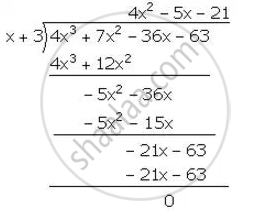Share

Using the Remainder Theorem, Factorise Each of the Following Completely. 4x3 + 7x2 – 36x – 63 - ICSE Class 10 - Mathematics

ConceptFactorising a Polynomial Completely After Obtaining One Factor by Factor Theorem

Question

Using the Remainder Theorem, factorise each of the following completely.

4x3 + 7x2 – 36x – 63

Solution

f(x)=4x^3+7x^2-36x-63

for x=3

f(x)=f(3)=4(3)^3+7(3)^2-36(3)-63

=108+63-108-63=0

Hence,(x+3) is a factor of f(x)∴ 4x^3+7x^2-36x-63=(x+3)(4x^2-5x-21)

= (x+3)(4x^2-12x+7x-21)

=(x+3)[4x(x-3)+7(x-3)]

=(x+3)(4x+7)(x-3)

Is there an error in this question or solution?

APPEARS IN

Solution Using the Remainder Theorem, Factorise Each of the Following Completely. 4x3 + 7x2 – 36x – 63 Concept: Factorising a Polynomial Completely After Obtaining One Factor by Factor Theorem.
S